Vibroengineering PROCEDIA

Published: 05 February 2022

# Effect of particle gradation on the triaxial test of soil using three-dimensional DEM analysis

Chenglong Jiang1
Chengshun Xu2
Kamran Iqbal3
1, 2, 3Key Laboratory of Urban Security and Disaster Engineering of Ministry of Education, Beijing University of Technology, Beijing, 100124, China
Corresponding Author:
Chengshun Xu
Views 38

#### Abstract

Numerical simulation of triaxial test are carried out for soil specimens with varying particle size by DEM analysis under the flexible boundary. For the purpose, soil state characteristics such as shear band formation, variation law and the difference between the global void ratio and the local void ratio are particularly focused. Results shows that the shear band of multi-graded specimen is more difficult to create, but it is thicker than that of single-graded specimen. Overall void ratio changes mostly due to particle movement at the shear band and independent to particle gradation. The shear strength of the multi-graded sample is better than that of the single-graded specimen.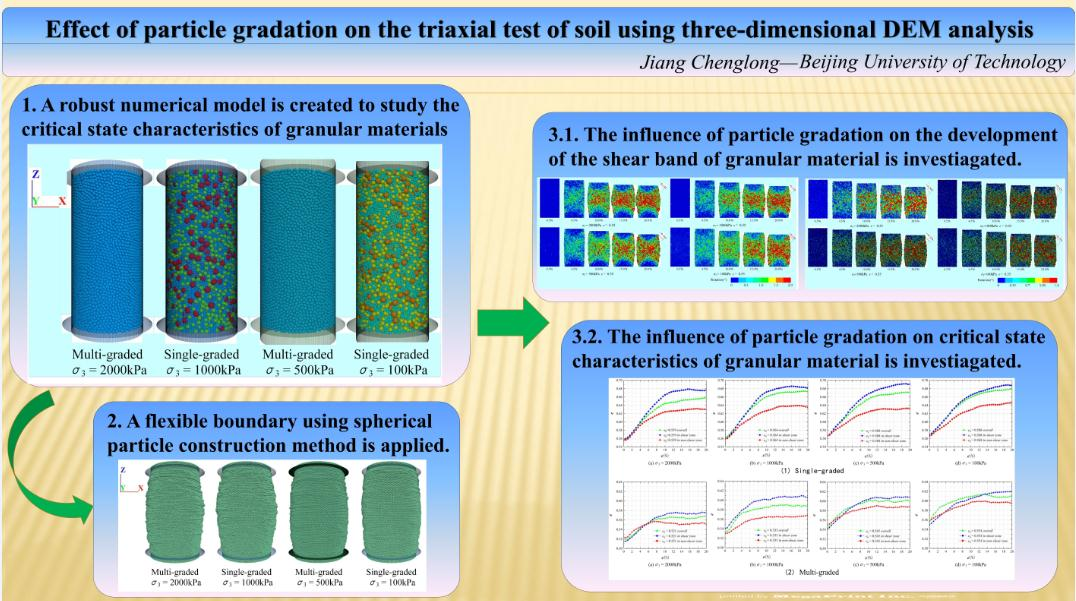#### Highlights

• A robust numerical model is created to study the critical state characteristics of granular materials.
• A flexible boundary using spherical particle construction method is applied.
• The influence of particle gradation on critical state characteristics of granular material is investiagated.

## 1. Introduction

Sand is a unique natural material with a wide range of properties that are difficult to predict. A structural failure will have a direct impact on the features of the mechanical behavior of sands. Density, water content, particle gradation are the most important soil structural features parameters. Particle size has been linked to the mechanical characteristics of sand in several studies -.

Experimental laboratory procedures are preferably used to investigate the mechanical characteristics of soil. Vallejo  studied the influence of particle gradation on the shear strength of unsaturated soil by testing the threshold value of soil strength variation with fine particles. By conducting laboratory tests, Jiang  studied the influence of particle gradation on soil’s compressive and tensile strength. Marachi  tested the strength of grading scale rockfill with different particle sizes usingtriaxial apparatus to investigate the influence of scale grade on the strength of rockfill. Li  found that greater the coarser particles, the higher the internal friction will be. Belkhatir  pointed out that the shear strength of sand increases with the increase of ${d}_{10}$ and ${d}_{50}$, but decreases with the increase of ${C}_{u}$.

It's impossible to precisely compute stress in the lab due to the infinite variable deformation of the sample after testing. It is worth to mention that the real void ratio in the shear band is different from one in the global, limiting the ability to accurately investigate mechanical behavior through laboratory experiments, since the void ratio determined in the stable condition is the specimen's overall void ratio.

When it comes to analyzing the meso-scale mechanics of materials with gradation, DEM is commonly employed as a micromechanical approach , , . Wood  proved that the critical state line of circular particles in $e$-$\mathrm{l}\mathrm{g}p$ plane, decreases with the widening of particles gradation by two-dimensional discrete element. Yan  obtained similar result from a three-dimensional perspective. Li ,  found that the critical state features of various graded soil specimens were influenced by the dimensions, shapes, and loading modes of the particles. Using the DEM, it is possible to better characterize granular materials' key state features.

In this research, a three-dimensional discrete element numerical simulation of the shear band creation and evolution, as well as the development of overall and local void ratios is used, to examine the impact of soil particle gradation on these parameters.

## 2. Establishment of discrete element numerical model for soil specimens

The numerical test plan is shown in Table 1 with two operating mode and eight groups. The cylindrical model has a diameter of 30 mm and height of 60 mm. When it comes to particle size classification, there are two options: single-graded and multi-graded. Models are built with an initial hypothetical void ratio of 0.55, and isotropic consolidation under confining pressures of 100, 500, 1000, and 2000 kPa is performed in order to obtain the actual void ratio; this is the initial void ratio ${e}_{0}$. The numerical simulation is divided into five steps: 1) Conventional spherical particles are used to simulate sand particles. According to the defined model size, the structural space composed of non-friction side boundary and upper and lower rigid loading surfaces to apply axial loading established to restrict the discrete granular material. 2) Based on the pre-determined parameters, the sand samples with two kinds of particle gradation were generated, as shown in Fig. 1. 3) The unbalanced force between particles is minimized through preliminary calculation to ensure that the particles of the sample are in close contact with each other when the model is completed. After that, each group of specimens are subjected to conventional isotropic servo consolidation through fish programming to make the soil reach the required physical state. 4) replace the conventional boundary with the flexible membrane boundary. Due to the possible soil disruption, constant confining pressure loading must be used to restore the specimen to its required consolidation state once the boundary conditions have been replaced. 5) the two-way load board and the membrane particles that are in contact with the loaded are equal, and the direction of the constant value is in the vertical direction of the specimen, In the loading phase, at the axial strain of 0.5 %, 1.5 %, 3.0 %, 4.5 %, 6.0 %, 10.0 %, 12.5 %, 15.0 %, 17.5 %, 20.0 % the cloud map of particle rotation, the void ratio of the local position and the macroscopic behaviors are recorded.

Table 1Parameters of the three-dimensional model for numerical simulation

 No# Granular level Consolidation pressure ${e}_{h}$ ${e}_{0}$ Particle grading (Volume) 1 Single-graded 2000 kPa 0.55 0.559 100 % ${r}_{a}$* 2 Multi-graded 0.521 30 % ${r}_{b}$, 40 % ${r}_{c}$, 30 %${r}_{d}$* 3 Single-graded 1000 kPa 0.564 100% ${r}_{a}$ 4 Multi-graded 0.533 30 % ${r}_{b}$, 40 % ${r}_{c}$, 30 % ${r}_{d}$ 5 Single-graded 500 kPa 0.568 100 % ${r}_{a}$ 6 Multi-graded 0.545 30 % ${r}_{b}$, 40 % ${r}_{c}$, 30 % ${r}_{d}$ 7 Single-graded 100 kPa 0.568 100 % ${r}_{a}$ 8 Multi-graded 0.554 30 % ${r}_{b}$, 40 % ${r}_{c}$, 30 % ${r}_{d}$ *${r}_{a}=$ 0.49-0.51 mm; ${r}_{b}=$ 0.4 mm; ${r}_{c}=$ 0.8 mm; ${r}_{d}=$ 1.2 mm

Fig. 1Meso-structure model of soils with different grain size distribution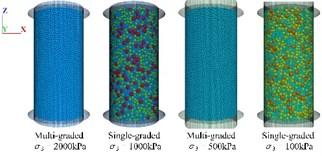Fig. 2Macroscopic simulation results of samples with different grain size distribution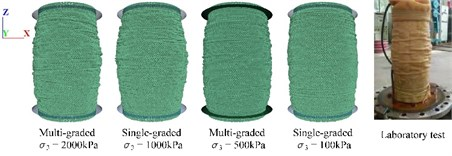According to the elastic theory of granular materials, the soil physical contact force transfer mode is determined by the contact model between particles, and the contact stiffness determines the soil elastic modulus. Therefore, the linear contact model is designated for the contact between sand particles, and the contact model between the flexible boundary particles is set to a linearcpond model. In order to prevent particle boundary fracture causing by the excessive concentrated force, the bond strength is set to a value of magnitude. The parameters selection of the contact model of specimens can be seen in Table 2.

Fig. 2 shows the results of the final failure mode of the numerical simulation with flexible membrane boundary and the laboratory test. It can be seen that the flexible boundary model can simulate the actual failure mode of laboratory test commendably.

Table 2Contact parameters between particles of sand

 Parameters Values Particle type Sample ball boundary ball Normal contact stiffness between particles (N/m) 5×106 2×104 Tangential contact stiffness between particles (N/m) 2×106 2×104 Interparticle friction coefficient 0.4 0.0 Local damping coefficient 0.7 – Particle density (kg/m3) 1700 600

## 3. Analysis of numerical simulation results

### 3.1. Analysis of formation and evolution of shear band of specimens

Fig. 3 shows the evolution nephogram of particle rotation of whole sand particles with two particle gradations under the same hypothetical void ratio (${e}_{h}=$ 0.55) and different consolidation conditions (100 kPa, 500 kPa, 1000 kPa, 2000 kPa) during shearing loading.

Fig. 3Sectional drawing of specimen particles with different axial strains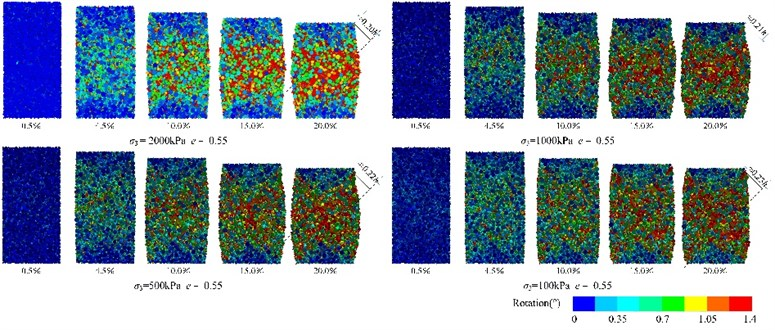a) Rotating nephogram of specimen particles with single-particle gradation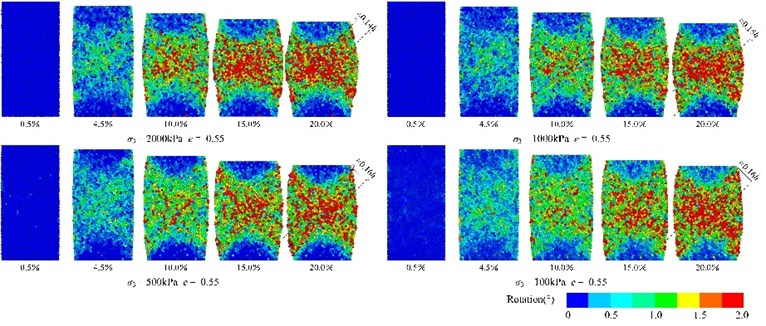b) Rotating nephogram of specimen particles with multi-particle gradation

## 4. Analysis of development of overall void ratio and local void ratio

Fig. 4 shows the development of the overall void ratio, the average void ratio in the shear band and the average void ratio in the non-shear region during the whole shearing loading of two groups of specimens with different particle gradations under four different consolidation conditions.

Fig. 4Comparison of variation of the overall void ratio and the local average void ratio of the specimens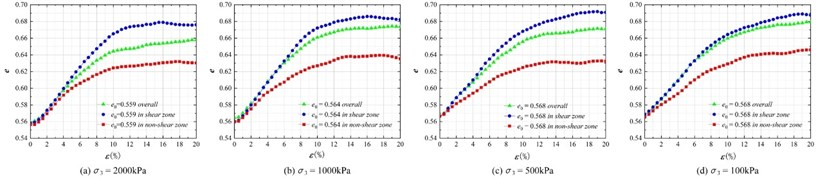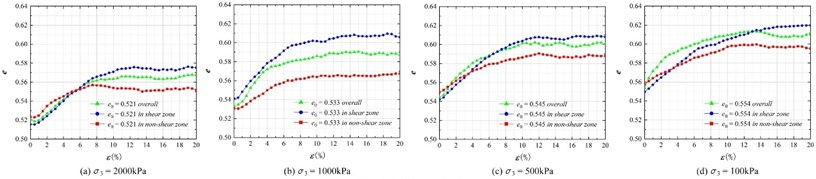It can be seen from the figure that: 1) when the axial strain reaches 20 %, the void ratio of the two groups of particle graded samples tends to be stable, indicating that the specimens enter the critical state. 2) During the shearing process, the variation amplitude of the overall void ratio of the two graded specimens is lower than the average void ratio in the shear band, but higher than the average void ratio outside the shear area. The development law of the overall void ratio is closer to the average void ratio in the shear band, indicating that the development of the global void ratio is largely dominated by the movement of particles in the shear zone, which can be explained from the particle rotation cloud diagram in Fig. 3. 3) The local void ratio of single particle size distribution specimen is similar in the mass, while the average void ratio of different parts of multi-graded sample is quite different. The variation ranges of the overall void ratio and the average void ratio inside and outside the shear region are lower for the multi-graded samples with the similar initial relative density. It shows that the extent of reaction of the overall void ratio of the simulation specimen is between the average void ratio inside and outside of the shear region. According to this law, the particle movement in the shear band dominates the total void ratio and has nothing to do with particle gradation. At the same time, the variation of the void ratio of multi-particle graded sand model is lower than that of the single graded specimen.

## 5. Analysis of stress-strain state

Fig. 5 shows all stress-strain curves of two kinds of soil specimens with particle grading under different consolidation conditions. It can be seen in the Fig. 5 that: 1) the partial stress of 8 groups of samples with different particle gradation and different consolidation states tends to be stable finally, indicating that the sand specimens with different gradation would get into the critical state. 2) The stress-strain relationship of sand specimens with single particle gradation presents obvious “softening” phenomenon under the consolidation state of 2000 kPa and 1000 kPa, while the stress-strain relationship of multi-graded specimens presents “hardening” as a whole. It shows that a sample with single-graded is easier to form a dense whole, while a multi graded model presents more local looseness under the condition of the similar initial density. The conclusion can also be drawn from the slow forming of the shear band in Fig. 4 and the inconsistency of the local void ratio as shown in Fig. 5. 3) The shear strength of multi-graded sand samples is higher than that of single-graded model, which is consistent with the laboratory test results.

Fig. 5Comparison of variation of the overall void ratio and the local average void ratio of the specimens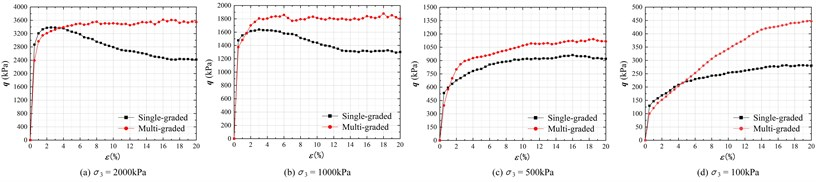## 6. Conclusions

In this paper, based on the flexible membrane boundary conditions, the numerical simulation of conventional triaxial test is carried out for soil specimens with different particle gradations. The influence of particle gradation on the formation and evolution of the shear band and the variation law of overall void ratio and local void ratio in the critical state is investigated. The main conclusions may be drawn as follow:

1) It is appropriate to describe the formation of the shear band in soil specimen by the rotation of sample particles. The specimens with two particle gradations show dilatancy failure under the flexible boundary condition, and the shear band formed after the shear failure of the specimens shows an asymmetric ‘X’ shape distribution. Due to the high local density, the particle rotation of the soil specimen with the single gradation in the shear band is large, and the difference between the particle rotation inside and outside the shear region is obvious. The thickness of the shear band of the two particle graded samples is related to the consolidation confining pressure conditions. Meanwhile the thickness of the shear band of the multi-graded specimen is significantly wider than that of the single-graded sample.

2) The increase amplitude of the overall void ratio of the specimen is between the change degree of the average void ratio inside and outside the shear region, and the change law is independent of the particle gradation and confining pressure of the sample in the numerical simulation. The local void ratio of the soil sample with multi-graded is non-uniform, and the variation amplitude is lower than that of the sample with single-graded specimen. The development of the overall void ratio of the sample with two kinds of gradation is dominated by the movement of particles in the shear band.

3) The influence of particle gradation on the strength and stress-strain mode of samples cannot be ignored in the simulation. The well graded specimen has higher shear strength, while the shear strength is lower of the mono-graded soil sample. The mono-graded specimens are homogeneous and dense as a whole, and the stress softening phenomenon of shear expansion is more likely to occur, while the multi particle graded samples show the stress hardening phenomenon under the same initial relative density owe to the regional in-homogeneity of the specimen itself.